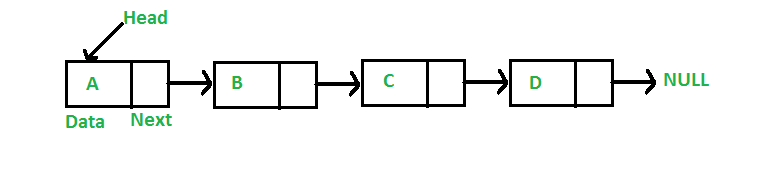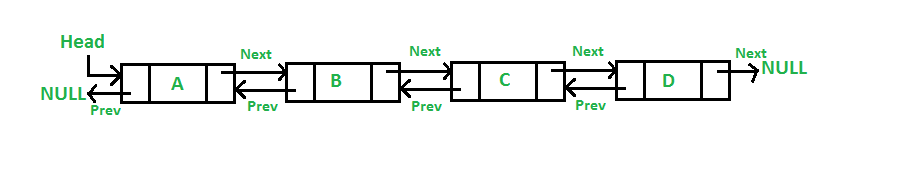Back

Product development

Our recently published article discussed the definition, importance, measurement method, and the types of time complexities in software engineering. Click here for a basic overview of data structures. As stated in the article mentioned earlier, measuring the efficiency of data structures is necessary to determine the use case of the different types of data structures.

## Types of Data Structures

Measuring the T(n) of accessing, searching, inserting, and deleting elements in a data structure, enables programmers in determining the suitable use of a specific data structure. While the efficiency does not dictate the use case of a data structure, it gives a good understanding of their behaviour. Highlighted below are some types of data structures:

### 1. Arrays and Array Lists Data Structures

Arrays are one of the most common types of data structures. They are essentially a list of similar elements stored in a central location. An array usually has a name used to reference it and a fixed size or length. The elements in an array hold the same type of information and are stored in sequential order on the computer memory. Each element is referenced by a numerical index which represents its position in the array. The first element has an index of 0, the second element index of 1, and goes on like that.

An ArrayList is similar to an Array, but differs in size. i.e. the  size of an ArrayList is subjected to changes, unlike an Array with a fixed size.

In some programming languages, Arrays and ArrayList are merged into a single data structure e.g., Lists in python and arrays in JavaScript. ArrayLists usually have built-in methods at disposal to access, search, delete, and insert elements. Arrays are often used where the size of the dataset is known and fixed an example being, a list containing data for each state in the country will always have a fixed size, while ArrayLists are used where the size can change for example, a list of followers of an Instagram account.

Measuring the Time Complexity of Arrays and ArrayLists

• Accessing an element has a T(n) of O(1). Elements can be accessed instantaneously by calling their index location. The size does not matter.

• Searching for an element has a T(n) of O(n). Arrays are mostly unsorted i.e., the elements are not in a numerical or some sort of order. This means taking into consideration the worst-case scenario, if the element to be found is the last one in the array, you may have to search through all the elements to find it.

• Inserting an element has a T(n) of O(n). Considering the worst-case scenario, if an element is to be inserted at index 0, every other element will have to be shifted to the right by one index. This gives a linear time complexity.

• Deleting an element has a T(n) of O(n). Deleting an element follows the same idea as inserting in arrays and ArrayLists. For the worst-case scenario, deleting the last element will cause every other element to be unshifted by one index.

### 2. Stacks

A stack is a sequential access data structure in which elements are added or removed based on the ‘Last In, First Out’, (LIFO) principle. This means the last element to be added will be the first to be retrieved or accessed. You can think of it as a stack (as the name implies) of plates. You can’t access a plate in the middle without possibly destroying the stack.

There is only one way in and out of the dataset. Stacks usually come with two methods built-in, which are the push and pop methods, used to push an object to the top of the stack and remove an object from the top of the stack respectively. Examples of scenarios stacks are most suitable for are undo/redo features of text editors, the back button on your browser, etc.

Measuring the Time Complexity of Stacks

• Accessing an element has a T(n) of O(n). This is because to access an element in the stack, you would have to pop off every element above it.

• Searching for an element has a T(n) of O(n). This is similar to accessing an element. To search for an element in the middle or end of the stack, you have to move through every element before it.

• Inserting an element has a T(n) of O(1). This is because the data flows in from a single point so adding an element can be done immediately.

• Deleting an element has a T(n) of O(1). This is also because the data flows in from a single point so deleting an element can be done immediately from the top.

### 3. Queues

A Queue is also a sequential access data structure in which elements are added or removed based on the ‘First In, First Out’, (FIFO) principle. This means the first element to be added will be the first to be retrieved or accessed. You can think of it as a line to use an ATM. The first person to arrive is the first person to use the machine. The later you arrive, the longer you wait. This means elements are added from the rear, known as the tail, and removed from the front, known as the head

Queues usually come with two methods built-in, which are the enqueue and dequeue methods, used to add an element to the tail of the queue and remove an element from the head of the queue respectively. Examples of scenarios stacks are most suitable for are job scheduling (the process in which the computer determines which task to complete for the user and when), by printers, when multiple documents are sent to know which to print next, etc.

Measuring the Time Complexity of Queues

• Accessing an element has a T(n) of O(n. Because accessing an element in the queue requires dequeuing every element preceding the one to be accessed.

• Searching for an element has a T(n) of O(n). The process of accessing elements is almost identical to  this. This is why retrieving an element in the middle or tail of a queue requires reviewing each element preceding it.
• Inserting an element has a T(n) of O(1). An Element in a queue can only be added at a single point – the tail.

• Deleting an element has a T(n) of O(1). Unlike inserting an element, deleting an element starts at a single point, which is at the head of the queue.

A LinkedList is a sequential access linear data structure where each element is an object called a node that contains two parts; the data, and the reference which points to the next node in the list. The data is where the actual content of the data structure is kept. The reference or pointer points to where the next node is stored in memory. A LinkedList starts with a node called the head node of the list and points to the second node and continues in that pattern. The last node called the tail node points to a null value.Application of LinkedLists includes being used as a backing of other data structures i.e., it can be used to make stacks, queues, etc. It is also used in the ‘up next’ feature of your music or movie player or a photo viewing software. It is important to note that the pointer of a node in a LinkedList can only point in one direction which is forward.

Measuring the Time Complexity of LinkedList

• Accessing an element has a T(n) of O(n). This is because a queue is a sequential access linear data structure. This means they can only be accessed in a distinct way. If we want to access an element, we would have to cycle through each node before it.

• Searching for an element has a T(n) of O(n). Searching is O(n) for the same reason. We will have to cycle through all the preceding nodes to find a node.

• Inserting an element has a T(n) of O(n) or O(1). To add an element to the end of a LinkedList, all you need to do is to point the reference of the last node to the new node and point the reference of the new node to null. To add an element at the beginning, the reference of the new node will be pointed to the head node of the list. To add an element to the middle of a LinkedList, you will have to point the node before the location to the new node and point the new node to the next node after the location. Inserting an element at the beginning or end of a LinkedList has an O(1) but inserting in the middle means we would have to traverse through the preceding nodes before inserting. This means sometimes inserting is O(1) and other times, O(n).

• Deleting an element has a T(n) of O(n) or O(1). To delete an element at the beginning, the reference of the head node will be pointed to null and that’s it,the node will be cut off from the rest of the list. To delete at the end of a LinkedList, set the pointer of the previous node to null instead of the current tail. To delete an element from the middle of a LinkedList, the node before the one to be removed is pointed to the node after the one to be removed. The process of deleting an element is identical to the process of inserting an element. Deleting at the beginning or ending will have a time complexity of O(1) while deleting an element in the middle will have a time complexity of O(n).